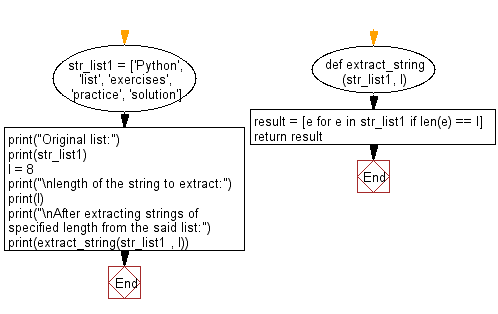﻿ Python: Extract specified size of strings from a give list of string values - w3resource# Python: Extract specified size of strings from a give list of string values

## Python List: Exercise - 102 with Solution

Write a Python program to extract specified size of strings from a give list of string values.

Sample Solution:

Python Code:

``````def extract_string(str_list1, l):
result = [e for e in str_list1 if len(e) == l]
return result

str_list1 = ['Python', 'list', 'exercises', 'practice', 'solution']
print("Original list:")
print(str_list1)
l = 8
print("\nlength of the string to extract:")
print(l)
print("\nAfter extracting strings of specified length from the said list:")
print(extract_string(str_list1 , l))
```
```

Sample Output:

```Original list:
['Python', 'list', 'exercises', 'practice', 'solution']

length of the string to extract:
8

After extracting strings of specified length from the said list:
['practice', 'solution']
```

Pictorial Presentation:Flowchart:## Visualize Python code execution:

The following tool visualize what the computer is doing step-by-step as it executes the said program:

Python Code Editor:

Have another way to solve this solution? Contribute your code (and comments) through Disqus.

What is the difficulty level of this exercise?

Test your Python skills with w3resource's quiz

﻿

## Python: Tips of the Day

```print(2_000_000)
```2000000